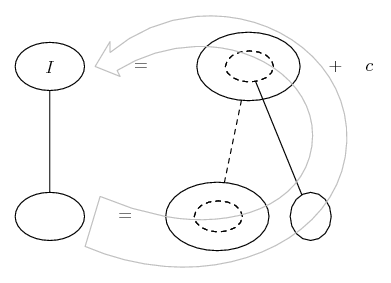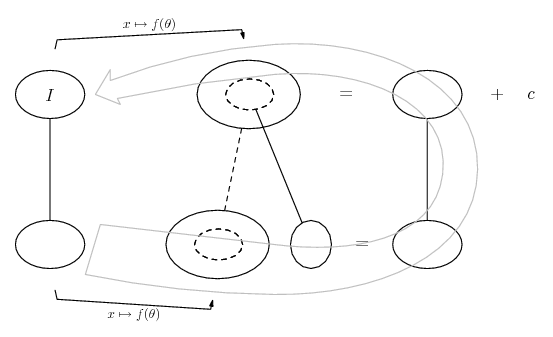# Thread: Help with integration

1. ##Help with integration

1) Integral {(x^3)-4x}/{(x^2)+1}^2.what Is The Solution?
2) Integral {x^(1/2)}dx/{1+ X^(1/4)}.what Is The Solution?

thaking you.

2.Originally Posted by ryanali1) Integral {(x^3)-4x}/{(x^2)+1}^2.what Is The Solution?
2) Integral {x^(1/2)}dx/{1+ X^(1/4)}.what Is The Solution?

thaking you.
1) Use partial fractions.

2) Substitute u = x^1/4.

If you need more help, please show your effort and say where you're stuck.

3. 1. $\displaystyle \displaystyle \int \frac{x^3-4x}{(x^2+1)^2}\;{dx}$ $\displaystyle \displaystyle = \int \frac{x(x^2-4)}{(x^2+1)^2}\;{dx}$

Let $\displaystyle \displaystyle t = x^2+1 \Rightarrow dx = \frac{1}{2x}\;{dt}$

Then also $\displaystyle x^2-4 = t-5$

We have $\displaystyle \displaystyle \int \frac{x^3-4x}{(x^2+1)^2}\;{dx}$ $\displaystyle \displaystyle = \frac{1}{2}\int \frac{x(t-5)}{xt^2}\;{dt} = \frac{1}{2}\int \left(\frac{1}{t}-\frac{5}{t^2}\right)\;{dt} = \ln{t}+\frac{5}{t}+k$

Hence $\displaystyle \displaystyle \int \frac{x^3-4x}{(x^2+1)^2}\;{dx} = \frac{(x^2+1)\ln(x^2+1)+5}{2(x^2+1)}+k$

4. Thank you very much to you both for replying.I tried the first using partial fractions and substitution,but got stuck and the second one i still have no idea how to go forward.
Thank you TheCoffeeMachine.If it is is'nt too much for you could you please help me with the second one too.

5. Just in case a picture helps...

In fact, a choice of two - either...... or...... where (key in spoiler) ...

Spoiler:... is the chain rule. Straight continuous lines differentiate downwards (integrate up) with respect to the main variable (in this case ), and the straight dashed line similarly but with respect to the dashed balloon expression (the inner function of the composite which is subject to the chain rule).

The general drift is...... or...Either way, work anti-clockwise, and find G then F then I.

_________________________________________

Don't integrate - balloontegrate!

Balloon Calculus; standard integrals, derivatives and methods

Balloon Calculus Drawing with LaTeX and Asymptote!

6.Originally Posted by ryanaliThank you very much to you both for replying.I tried the first using partial fractions and substitution,but got stuck and the second one i still have no idea how to go forward.
Thank you TheCoffeeMachine.If it is is'nt too much for you could you please help me with the second one too.
You should post all the work you have done and then say specifically where you are stuck.

#### Search Tags

integration